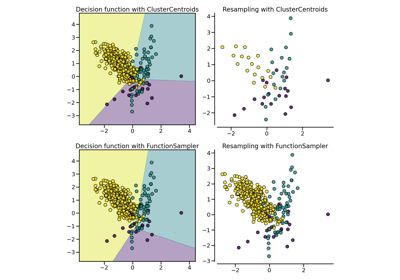# `imblearn.under_sampling`.CondensedNearestNeighbour¶

class `imblearn.under_sampling.``CondensedNearestNeighbour`(*, sampling_strategy='auto', random_state=None, n_neighbors=None, n_seeds_S=1, n_jobs=None)[source]

Undersample based on the condensed nearest neighbour method.

Read more in the User Guide.

Parameters
sampling_strategystr, list or callable

Sampling information to sample the data set.

• When `str`, specify the class targeted by the resampling. Note the the number of samples will not be equal in each. Possible choices are:

`'majority'`: resample only the majority class;

`'not minority'`: resample all classes but the minority class;

`'not majority'`: resample all classes but the majority class;

`'all'`: resample all classes;

`'auto'`: equivalent to `'not minority'`.

• When `list`, the list contains the classes targeted by the resampling.

• When callable, function taking `y` and returns a `dict`. The keys correspond to the targeted classes. The values correspond to the desired number of samples for each class.

random_stateint, RandomState instance, default=None

Control the randomization of the algorithm.

• If int, `random_state` is the seed used by the random number generator;

• If `RandomState` instance, random_state is the random number generator;

• If `None`, the random number generator is the `RandomState` instance used by `np.random`.

n_neighborsint or object, default= KNeighborsClassifier(n_neighbors=1)

If `int`, size of the neighbourhood to consider to compute the nearest neighbors. If object, an estimator that inherits from `sklearn.neighbors.base.KNeighborsMixin` that will be used to find the nearest-neighbors.

n_seeds_Sint, default=1

Number of samples to extract in order to build the set S.

n_jobsint, default=None

Number of CPU cores used during the cross-validation loop. `None` means 1 unless in a `joblib.parallel_backend` context. `-1` means using all processors. See Glossary for more details.

`EditedNearestNeighbours`

Undersample by editing samples.

`RepeatedEditedNearestNeighbours`

Undersample by repeating ENN algorithm.

`AllKNN`

Undersample using ENN and various number of neighbours.

Notes

The method is based on .

Supports multi-class resampling. A one-vs.-rest scheme is used when sampling a class as proposed in .

References

1(1,2)

P. Hart, “The condensed nearest neighbor rule,” In Information Theory, IEEE Transactions on, vol. 14(3), pp. 515-516, 1968.

Examples

```>>> from collections import Counter
>>> from sklearn.datasets import fetch_mldata
>>> from imblearn.under_sampling import CondensedNearestNeighbour
>>> pima = fetch_mldata('diabetes_scale')
>>> X, y = pima['data'], pima['target']
>>> print('Original dataset shape %s' % Counter(y))
Original dataset shape Counter({1: 500, -1: 268})
>>> cnn = CondensedNearestNeighbour(random_state=42)
>>> X_res, y_res = cnn.fit_resample(X, y)
>>> print('Resampled dataset shape %s' % Counter(y_res))
Resampled dataset shape Counter({-1: 268, 1: 227})
```
Attributes
sample_indices_ndarray of shape (n_new_samples)

Indices of the samples selected.

New in version 0.4.

`__init__`(self, *, sampling_strategy='auto', random_state=None, n_neighbors=None, n_seeds_S=1, n_jobs=None)[source]

Initialize self. See help(type(self)) for accurate signature.

## Examples using `imblearn.under_sampling.CondensedNearestNeighbour`¶